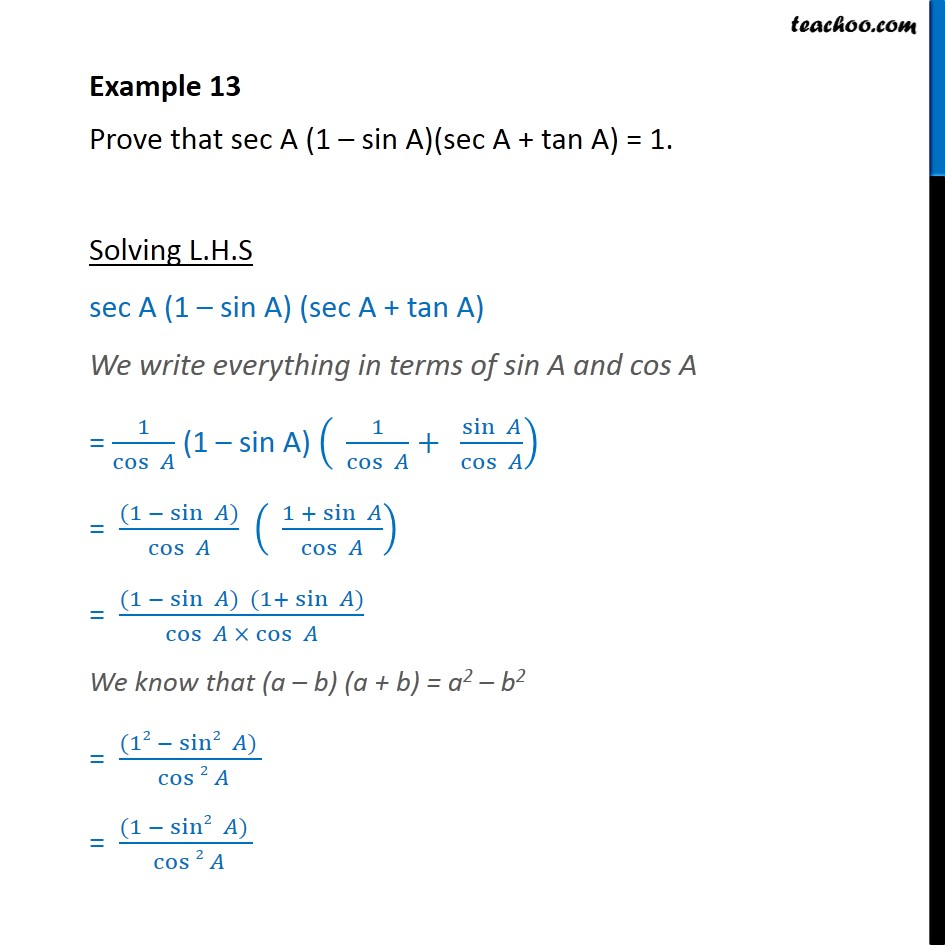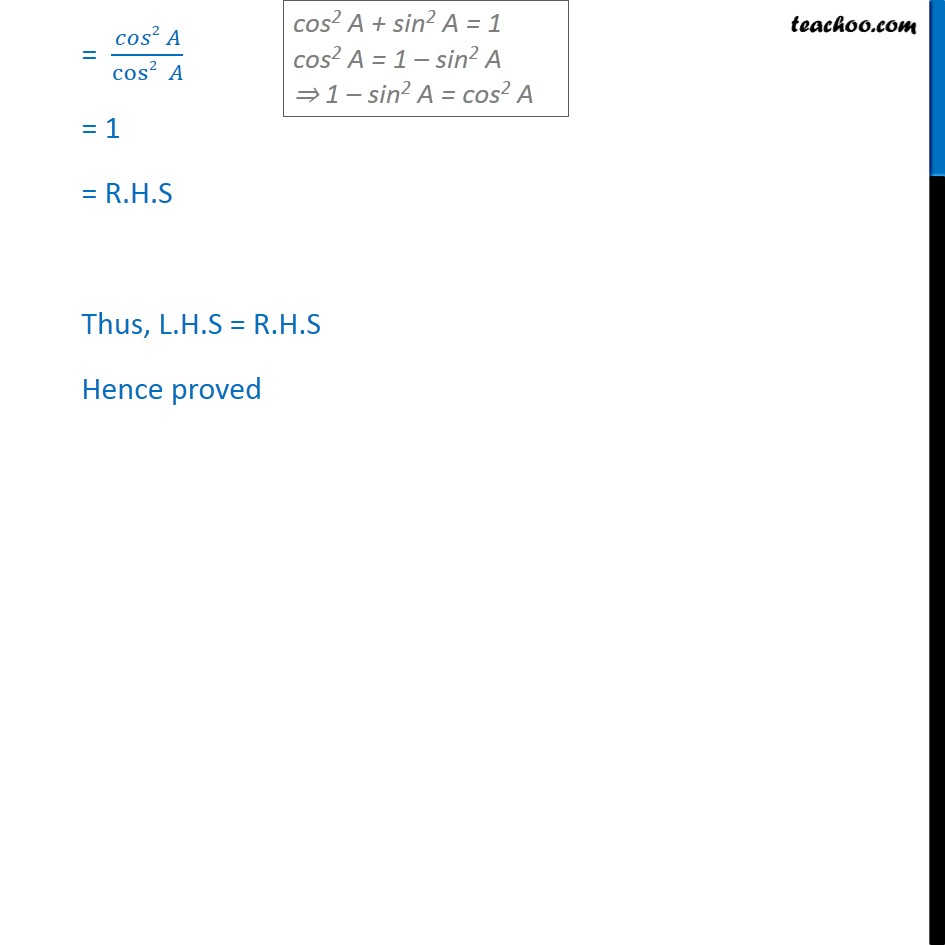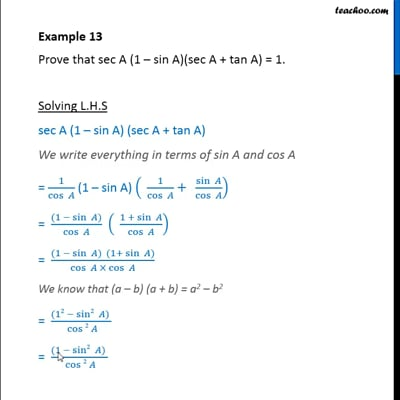Evaluating using Trignometric Identities

Chapter 8 Class 10 Introduction to Trignometry
Concept wiseThis video is only available for Teachoo black users

Introducing your new favourite teacher - Teachoo Black, at only ₹83 per month

### Transcript

Example 13 Prove that sec A (1 sin A)(sec A + tan A) = 1. Solving L.H.S sec A (1 sin A) (sec A + tan A) We write everything in terms of sin A and cos A = 1/cos (1 sin A) (" " 1/cos +" " sin /cos ) = ((1 sin ) )/(cos ) (" " (1 + sin )/cos ) = ((1 sin ) (1+ sin ) )/(cos cos ) We know that (a b) (a + b) = a2 b2 = ((12 sin2 ) )/( cos 2 ) = ((1 sin2 ) )/( cos 2 ) = ( 2 )/(cos2 ) = 1 = R.H.S Thus, L.H.S = R.H.S Hence proved